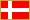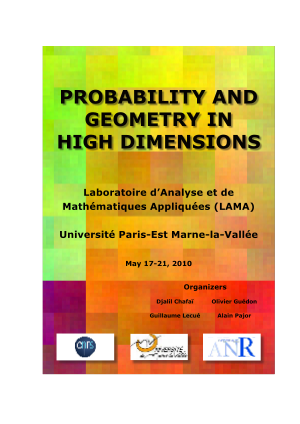# Month: May 2010

Let $(T_n)_{n\geq0}$ be the Chebyshev polynomials of the first kind given by

$\displaystyle T_n(\cos(x))=\cos(nx).$

These polynomials are orthogonal with respect to the arcsine probability distribution

$\displaystyle x\mapsto \frac{1}{\pi\sqrt{1-x^2}}\mathbf{1}_{[-1,1]}(x).$

They satisfy to the recurrence relation $T_0=1$, $T_1(x)=x$ and

$\displaystyle T_{n+1}(x)=2xT_n(x)-T_{n-1}(x).$

First Haagerup formula: if $-2\leq x\neq y\leq 2$ then (the series is convergent)

$\displaystyle \log\left|x-y\right|=-\sum_{n=1}^{\infty}\frac{2}{n}T_n\left(\frac{x}{2}\right)T_n\left(\frac{y}{2}\right).$

Second Haagerup formula: if $x>2$ and $-2\leq y\leq 2$ then (absolutely convergent series)

$\displaystyle \log\left|x-y\right|=\log\left|\frac{x+\sqrt{x^2-4}}{2}\right|-\sum_{n=1}^\infty\frac{2}{n}\left(\frac{x-\sqrt{x^2-4}}{2}\right)^nT_n\left(\frac{y}{2}\right).$

I have learnt these beautiful formulas in a talk given by Ionel Popescu during the Workshop Probability and Geometry in High Dimensions held at Marne-la-Vallée. The proofs are elementary. These formulas are deeply related to the fact that the arcsine distribution  on $[-a,a]$ is the maximum of the Voiculescu entropy (i.e. minimum of logarithmic energy) over the set of probability distributions supported in $[-a,a]$. This fact is quite classical, and goes back at least to the works of  Erdős and Turán , and Szegő, on the equilibrium measure of the roots of orthogonal polynomials. You may take a look at the books by Saff and Totik and by van Assche.Note: Uffe Haagerup is a Danish mathematician. His MR number is 78865.

Last Updated on 2019-11-10

In terms of energy, the Métro is an oriented weighted graph, due to the presence of stairs.Last Updated on 2010-06-14

I realized this week that my former PhD advisor has spent a significant amount of his  research time juggling with the Talagrand $\gamma_2$ and the Bakry-Émery $\Gamma_{\!\!2}$. He belongs to this very small set of individuals versed in these two universes, between analysis, geometry, and probability. The link is clearly the Gaussian law. It might be amusing to connect the two in a deep statement.

Recently, a kind of Bakry-Émery $\Gamma_2$ was developed for Markov chains on metric spaces.

More recently, the Talagrand $\gamma_2$ was used successfully for random walks on graphs.

Last Updated on 2019-11-10

We organize with my colleagues Olivier Guédon, Guillaume Lecué, and Alain Pajor, of Marne-la-Vallée, a one week workshop on Probability & Geometry in High Dimensions.

The aim of this workshop is to reflect on recent developments in Probability and Geometry in High Dimensions with emphasis on interactions with other fields of mathematics such as compressed sensing, sparse statistical problems, random matrices, and empirical processes.

The workshop will take place on the campus of Université Paris-Est Marne-la-Vallée, May 17-21 2010. It turns out that Michel Talagrand will give a talk at this occasion.Syntax · Style · .